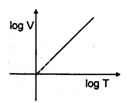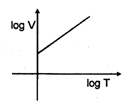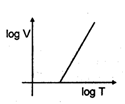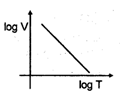What would be the final pressure of O2 in the following experiment? A collapsed polythene bag of 30 litre capacity is partially blown up by the addition of 10 litre of N2 at 0.965 atm at 298 K. Subsequently,  enough O2  is pumped into bag so that at 298 K and external pressure of 0.990 atm, the bag contains full 30 litre.

1. 0.67 atm
2. 0.52 atm
3. 85 atm
4. 20 atm

Concept Questions :-

Ideal Gas Law
High Yielding Test Series + Question Bank - NEET 2020

Difficulty Level:

A 40 mL of a mixture of H2 and O2 was placed in a gas burette at 18 oC and  1 atm pressure. A spark was supplied so that the formation of water was complete . The remaining pure gas had a volume of 10 mL at 18 oC  and 1 atm pressure. If the remaining gas was H2 , what was the initial mole percentage of H2 in the mixture?

1.  25%

2.  50%

3.  35%

4.  75%

Concept Questions :-

Gas Law
High Yielding Test Series + Question Bank - NEET 2020

Difficulty Level:

60 mL of a mixture of equal volume of Cl2 and an oxide of chlorine was heated and then cooled back to the original temperature. The resulting gas mixture was found to have volume of 75 ml. On treatment with caustic soda solution, the volume contracted to 15 ml. Assume that all the measurements are made at same T and P. Deduce the simplest formula for oxide of Cl2. The oxide of Cl2 on heating decomposes quantitatively to O2 and Cl2.

1. ClO
2. Cl2O
3. ClO4
4. Cl2O3
High Yielding Test Series + Question Bank - NEET 2020

Difficulty Level:

For the reaction, N2 O(g)2NO2 (g) + 0.5 O2 (g),

Calculate the mole fraction of N2 O5 (g) decomposed at a constant volume and temperature if the final pressure is 600 mm Hg and the pressure at any time is 960 mm Hg.  Assume ideal gas behaviour.

1. 0.4
2. 0.2
3. 0.1
4. 0.5

Concept Questions :-

Dalton's Law
High Yielding Test Series + Question Bank - NEET 2020

Difficulty Level:

An underwater bubble with radius of  5 cm at the bottom of tank , where the temperature is 5oC and pressure is 3 atm rises to the surface, where temperature is 25 oC and pressure is 1 atm. What will be the radius of bubble when it reaches to the surface?

1. 7.4 cm
2. 4.9 cm
3. 9.4 cm
4. 13 cm
High Yielding Test Series + Question Bank - NEET 2020

Difficulty Level:

If density of vapours of a substance of molar mass 18 gm / mole at 1 atm pressure and 500 K is 0.36 kg m–3 , then value of Z for the vapours is : (Take R = 0.082 L atm mole K–1)

(1) $\frac{41}{50}$

(2) $\frac{50}{41}$

(3) 1.1

(4) 0.9

Concept Questions :-

Compressibility Factor
High Yielding Test Series + Question Bank - NEET 2020

Difficulty Level:

Equal amount (mass) of methane and ethane have their total translational kinetic energy in the ratio

3: 1 then their temperatures are in the ratio.

(1) 5 : 8

(2) 45 : 8

(3) 15 : 8

(4) 8 : 5

Concept Questions :-

Kinetic Theory of Gas
High Yielding Test Series + Question Bank - NEET 2020

Difficulty Level:

Which of the following sketches is an isobar $\left(\frac{\mathrm{nR}}{\mathrm{p}}\mathrm{ }>\mathrm{ 1}\right)$

(1)(2)(3)(4)Concept Questions :-

Ideal Gas Law
High Yielding Test Series + Question Bank - NEET 2020

Difficulty Level:

For a certain gas which deviates a little from ideal behaviour, the values of density, p

were measured at different values of pressure, P. The plot of P/p on the Y–axis versus P on the X–axis was nonlinear and had an intercept on the Y–axis, which was equal to

(1) $\frac{\text{RT}}{\text{M}}$

(2) $\frac{\text{M}}{\text{RT}}$

(3) RT

(4) $\frac{\text{RT}}{\text{V}}$

Where M = molar mass.

Concept Questions :-

Ideal Gas Law
High Yielding Test Series + Question Bank - NEET 2020

Difficulty Level:

A sample of water gas has a composition by volume of 50% H2, 45% CO and 5% CO2. Calculate the volume in litre at S.T.P. of water gas which on treatment with excess of stream will produce 5 litre H2. The equation for the reaction is : CO + H2O $\stackrel{}{\to }$ CO2+ H2

(1) 4.263 Litre

(2) 5.263 Litre

(3) 6.263 Litre

(4) 7.263 Litre

Concept Questions :-

Gas Law